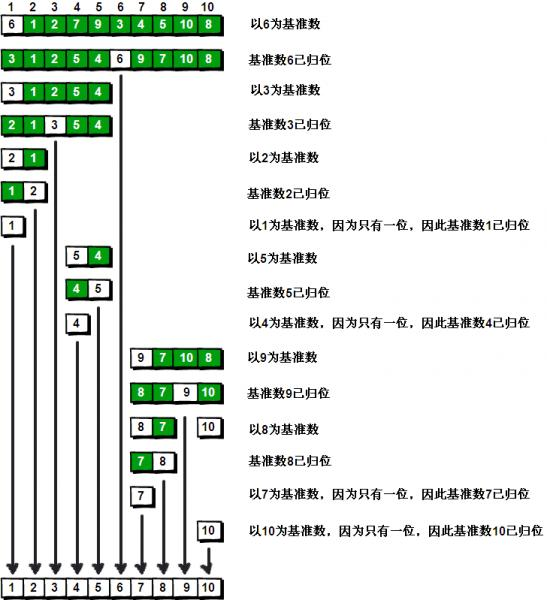## 给定一个N×N的二维矩阵表示图像，90度顺时针旋转图像。

### 样例

• 先上下翻， 以X轴对称
• 再调换坐标
```public class Solution {
/**
* @param matrix: a lists of integers
* @return: nothing
*/
public void rotate(int[][] matrix) {

int l = matrix.length;
for (int i = 0; i < l / 2; i++) {
for (int j = i; j < l - 1 - i; j++) {
int tmp = matrix[i][j];
matrix[i][j] = matrix[l - 1 - j][i];
matrix[l - 1 - j][i] = matrix[l - 1 - i][l - 1 - j];
matrix[l - 1 - i][l - 1 - j] = matrix[j][l - 1 - i];
matrix[j][l - 1 - i] = tmp;

}
}

for (int[] _n : matrix) {
System.out.print("[");
for (int x : _n) {
System.out.print(x + ",");
}
System.out.print("]\n");
}

}
}```

## 快排算法的实现与讲解(java/C++)

6 >= 5 条件成立，接着左边往右边移动一位（j–）

……

4>=5条件不成立，这个时候就换一下位置，4跟5换。现在的数组应该就是这样子：{4,2,1,8,9,3,7,0,5,6}

5>=4条件成立，右边往左边靠拢（i++）

5>=2条件成立，右边往左边靠拢（i++）

……

5>=8条件不成立，换位置：{4,2,1,5,9,3,7,0,8,6}

{4,2,1,0,9,3,7,5,8,6}

{4,2,1,0,5,3,7,9,8,6}

….以此类推，哨兵i继续向右移动，悲剧！！！此时i和j撞上了，说明此时“探测”结束。我们将基准数5和3进行交换。交换之后的序列如下：

{4,2,1,0,3,5,7,9,8,6}

{4,2,1,0,3,        5       ,7,9,8,6}• 快排的原理很简单；
• 就是把数组分为两节；
• 左边的是最小的，而右边的是最大的；
• 然后再拿左、右边的来继续递归，递归的原理也一样，也是拆分为两节，以此类推。

2.java代码:

```public static void main(String[] args) {
int[] arr = {5,2,1,8,9,3,7,0,4,6};
sort(arr, 0, arr.length - 1);
for (int i : arr) {
System.out.print(i + " ");
}
}

private static void sort(int[] arr, int l, int r) {
int i = l;
int j = r;
if (l < r) {
while (l < r) {
while (l < r && arr[r] >= arr[l]) {
r--;
}
int tmp = arr[l];
arr[l] = arr[r];
arr[r] = tmp;

while (l < r && arr[l] <= arr[r]) {
l++;
}
tmp = arr[l];
arr[l] = arr[r];
arr[r] = tmp;

}
sort(arr, i, l - 1);//递归左边，此时l=5
sort(arr, l + 1, j);//递归右边，此时l=5
}
}```

2.那么c++的代码会是怎么样呢？

```#include "pch.h"
#include <iostream>

using namespace std;

void sortQ(int *arr, int l, int r) {
int i = l;
int j = r;
int tmp;
if (i < j) {
while (l < r) {
while (l < r && arr[r] >= arr[l])
{
r--;
}

tmp = arr[l];
arr[l] = arr[r];
arr[r] = tmp;

while (l < r && arr[r] >= arr[l]) {
l++;
}
tmp = arr[l];
arr[l] = arr[r];
arr[r] = tmp;
}

sortQ(arr, i, l - 1);//处理左边，此时l=5
sortQ(arr, l + 1, j);//处理右边，此时l=5
}

}

int main()
{
std::cout << "Hello World!\n";

int arr[] = {5,2,1,8,9,3,7,0,4,6};
int size = sizeof(arr) / sizeof(arr);

sortQ(arr, 0, size - 1);
for (int i : arr) {
cout << i << " ";
}

}```

1.Document

2.SAXParser

SAXParser是一个用于处理XML的事件驱动的“推”模型。它不是W3C标准，但它是一个得到了广泛认可的API，大多数SAXParser解析器在实现的时候都遵循标准。

SAXParser解析器不象DOM那样建立一个整个文档的树型表示，而是使用数据流的方式读取，然后根据读取文档的元素类型进行事件反馈。这些事件将会推给事件处理器，而事件处理器则提供对文档内容的访问数据包装等。

## FileChannel文件通道

FileChannel

FileChannel 可以通过 RandomAccessFile、FileInputStream、FileOutStream也可以通过FileChannel.open 获取。

write 和 append 有什么区别?

FileChannel.lock tryLock 从文档上看一个是同步阻塞、另一个是非阻塞。

tryLock 在同一个JVM中不同线程获取时，先到先得，后到的返回null，但我在windows上测试为抛出异常:OverlappingFileLockException ，据说 Linux 上抛出【java.io.IOException:Permission denied】。

tryLock 和 lock 都提供一个API含有三个参数

lock(long position,
long size,
boolean shared)

lock(long position,
long size,
boolean shared) // 是否共享锁

FileLock.isShared()

while (true) {
try {
lock = fc.lock();
break;
} catch (OverlappingFileLockException e) {
}
}

```import java.io.FileInputStream;
import java.io.FileNotFoundException;
import java.io.FileOutputStream;
import java.io.IOException;
import java.nio.ByteBuffer;
import java.nio.MappedByteBuffer;
import java.nio.channels.FileChannel;
import java.nio.file.Path;
import java.nio.file.Paths;

/**
* Created by alan on 2018/12/15.
*/
public class FileChannelDemo extends OutPut {

public static void main(String[] args) {
String path = "d:/1.txt";
w(path);
try {
Path paths = Paths.get(path);
//            FileChannel channel = FileChannel.open(paths);//HEX{01 02 03 04}
FileInputStream in = new FileInputStream(path);
FileChannel channel = in.getChannel();
MappedByteBuffer buffer = channel.map(FileChannel.MapMode.READ_ONLY, 0, channel.size());

String str = "";
for (int i = 0; i < channel.size(); i++) {
str += buffer.get() + " ";
}
out(str);

buffer.flip();
channel.close();
in.close();

} catch (IOException e) {
e.printStackTrace();
}

}

public static void w(String path) {
try {

FileOutputStream out = new FileOutputStream(path);
FileChannel channel = out.getChannel();
ByteBuffer buffer = ByteBuffer.allocate(20);

buffer.put((byte) 0x1B);
buffer.put((byte) 0x2B);
buffer.put((byte) 0x3B);
buffer.put((byte) 0x4B);
buffer.put((byte) 0x5B);
buffer.put((byte) 0xFB);
buffer.put((byte) 0xEB);

buffer.flip();

channel.write(buffer,0);
channel.close();

out.flush();
out.close();
} catch (FileNotFoundException e) {
e.printStackTrace();
} catch (IOException e) {
e.printStackTrace();
}
}

}```